Tamilnadu State Board New Syllabus Samacheer Kalvi 8th Maths Guide Pdf Chapter 2 Measurements Ex 2.1 Text Book Back Questions and Answers, Notes.

## Tamilnadu Samacheer Kalvi 8th Maths Solutions Chapter 2 Measurements Ex 2.1

Question 1.
Fill in the blanks:
(i) The ratio between the circumference and diameter of any circle is _______ .
π(ii) A line segment which joins any two points on a circle is a _______ .
Chord

(iii) The longest chord of a circle is _______ .
Diameter

(iv) The radius of a circle of diameter 24 cm is _______ .
12 cm

(v) A part of circumference of a circle is called as _______ .
an arcQuestion 2.
Match the following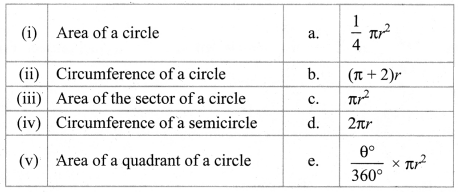(i) – c
(ii) – d
(iii) – e
(iv) – b
(v) – a

Question 3.
Find the central angle of the shaded sectors (each circle is divided into equal sectors).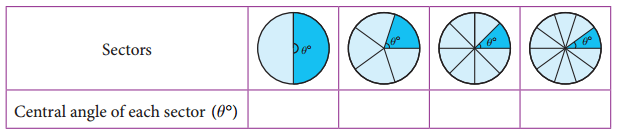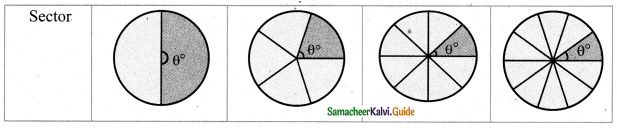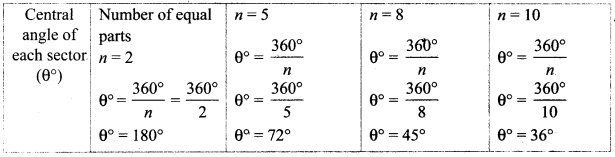Question 4.
For the sectors with given measures, find the length of the arc, area and perimeter.
(π = 3. 14)
(i) central angle 45° r = 16 cm
(i) central angle 45° r = 16 cm
Length of the arc l = $$\frac{\theta^{\circ}}{360^{\circ}}$$ × 2πr units
l = $$\frac{45^{\circ}}{360^{\circ}}$$ × 2 × 3.14 × 16 cm
l = $$\frac{1}{8}$$ × 2 × 3.14 × 16 cm
l = 12.56 cm
Area of the sector = $$\frac{\theta^{\circ}}{360^{\circ}}$$ × πr2 sq. units
A = $$\frac{45^{\circ}}{360^{\circ}}$$ × 3.14 × 16 × 16
A = 100.48 cm2
Perimeter of the sector P = l + 2r units
P = 12.56 + 2(16) cm
p = 44.56 cm

(ii) central angle 120°, d = 12.6 cm
∴ r = $$\frac{12.6}{2}$$ cm
r = 6.3cm
Length of the arc l = $$\frac{\theta^{\circ}}{360^{\circ}}$$ × 2πr units
l = $$\frac{120^{\circ}}{360^{\circ}}$$ × 2 × 3.14 × 63 cm
l = 13.188cm
I = 13.19cm
Area of the sector A = $$\frac{\theta^{\circ}}{360^{\circ}}$$ × πr2 sq. units
A = $$\frac{120^{\circ}}{360^{\circ}}$$ × 3 14 × 6.3 × 6.3 cm2
A = 3.14 × 6.3 × 2.1 cm2
A = 41.54 cm2
Perimeter of the sector P = l + 2r cm
P = 13.19 + 2(6.3) cm
= 13.19 + 1.2.6 cm
P = 25.79 cmQuestion 5.
From the measures given below, find the area of the sectors.
(i) Length of the arc = 48 m, r = 10 m
Area of the sector A = $$\frac{l r}{2}$$ sq. units
l = 48m
r = 10m
= $$\frac{48 \times 10}{2}$$ m2
= 24 × 10m2
= 240 m2
Area of the sector = 240 m2

(ii) length of the arc = 50 cm, r = 13.5 cm
Length of the arc l = 12.5 cm
Area of the sector A = $$\frac{l r}{2}$$ sq. units
A = $$\frac{12.5 \times 6}{2}$$
A = 12.5 × 3cm2
A = 37.5 cm2
Area of the sector A = 37.5 cm2Question 6.
Find the central angle of each of the sectors whose measures are given below. (π = $$\frac{22}{7}$$)
(i) area = 462 cm2, r = 21 cm
area = 462 cm2, r = 21 cm
Radius of the Sector = 21 cm
Area of the sector = 462 cm2
$$\frac{l r}{2}$$ = 462
$$\frac{l \times 21}{2}$$ = 462
l = $$\frac{462 \times 2}{21}$$
l = 22 × 2
Length of the arc l = 44 cm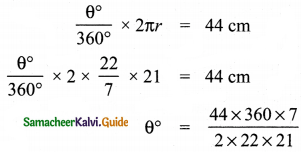θ° = 120°
∴ Central angle of the sector = 120°

(ii) length of the arc = 44 m, r = 35 m
Radius of the sector = 8.4cm
Area of the sector = 18.48 cm2
$$\frac{l r}{2}$$ = 18.48
$$\frac{1 \times 8.4}{2}$$ = 18.48
l = $$\frac{18.48 \times 2}{8.4}$$
Hint:Length of the arc l = 4.4 cm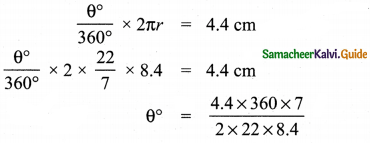Hint:θ° = 30°
Central angle = 30°Question 7.
A circle of radius 120 m is divided into 8 equal sectors. Find the length of the arc of each of the sectors.
Radius of the circle r = 120 m
Number of equal sectors = 8
∴ Central angle of each sector = $$\frac{360^{\circ}}{n}$$
θ° = $$\frac{360^{\circ}}{8}$$
θ° = 45°
Length of the arc l = $$\frac{\theta^{\circ}}{360^{\circ}}$$ × 2πr units
= $$\frac{45^{\circ}}{360^{\circ}}$$ × 2π × 120 m
Length of the arc = 30 × πm

Another method:
l = $$\frac{1}{n}$$ × 2πr = $$\frac{1}{8}$$ × 2 × π × 120 = 30 π m
Length of the arc = 30 π m

Question 8.
A circle of radius 70 cm is divided into 5 equal sectors. Find the area of each of the sectors.
Radius of the sector r = 70 cm
Number of equal sectors = 5
∴ Central angle of each sector = $$\frac{360^{\circ}}{n}$$
θ° = 360°
θ° = 72°
Area of the sector = $$\frac{\theta^{\circ}}{360^{\circ}}$$ × πr2 sq.units
= $$\frac{72^{\circ}}{360^{\circ}}$$ × π × 70 × 70cm2
Hint:= 14 × 70 × πcm2
= 980 πcm2
Note: We can solve this problem using A = $$\frac{1}{n}$$ πr2 sq. units also.Question 9.
Dhamu fixes a square tile of 30cm on the floor. The tile has a sector design on it as shown in the figure. Find the area of the sector. (π = 3.14).Side of the square = 30 cm
∴ Radius of the sector design = 30 cm
Given the design of a circular quadrant.
Area of the quadrant = $$\frac{1}{4}$$ πr2 sq.units
= $$\frac{1}{4}$$ × 3.14 × 30 × 30cm2
= 3.14 × 15 × 15cm2
∴ Area of the sector design = 706.5 cm2 (approximately)Question 10.
A circle is formed with 8 equal granite stones as shown in the figure each of radius 56 cm and whose central angle is 45°. Find the area of each of the granite stones. (π = $$\frac { 22 }{ 7 }$$)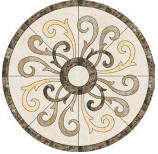Area of each sector = $$\frac{1}{n}$$ πr2 sq. units
= $$\frac{1}{8} \times \frac{22}{7}$$ × 56 × 56cm2 =1232 cm2